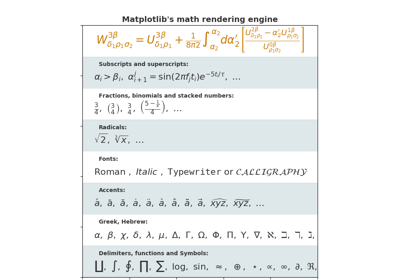You are reading an old version of the documentation (v3.0.0). For the latest version see https://matplotlib.org/stable/api/_as_gen/matplotlib.pyplot.fill_between.html

# matplotlib.pyplot.fill_between¶

`matplotlib.pyplot.``fill_between`(x, y1, y2=0, where=None, interpolate=False, step=None, *, data=None, **kwargs)[source]

Fill the area between two horizontal curves.

The curves are defined by the points (x, y1) and (x, y2). This creates one or multiple polygons describing the filled area.

You may exclude some horizontal sections from filling using where.

By default, the edges connect the given points directly. Use step if the filling should be a step function, i.e. constant in between x.

Parameters:
x : array (length N)

The x coordinates of the nodes defining the curves.

y1 : array (length N) or scalar

The y coordinates of the nodes defining the first curve.

y2 : array (length N) or scalar, optional, default: 0

The y coordinates of the nodes defining the second curve.

where : array of bool (length N), optional, default: None

Define where to exclude some horizontal regions from being filled. The filled regions are defined by the coordinates `x[where]`. More precisely, fill between `x[i]` and `x[i+1]` if `where[i] and where[i+1]`. Note that this definition implies that an isolated True value between two False values in where will not result in filling. Both sides of the True position remain unfilled due to the adjacent False values.

interpolate : bool, optional

This option is only relvant if where is used and the two curves are crossing each other.

Semantically, where is often used for y1 > y2 or similar. By default, the nodes of the polygon defining the filled region will only be placed at the positions in the x array. Such a polygon cannot describe the above semantics close to the intersection. The x-sections containing the intersection are simply clipped.

Setting interpolate to True will calculate the actual intersection point and extend the filled region up to this point.

step : {'pre', 'post', 'mid'}, optional

Define step if the filling should be a step function, i.e. constant in between x. The value determines where the step will occur:

• 'pre': The y value is continued constantly to the left from every x position, i.e. the interval `(x[i-1], x[i]]` has the value `y[i]`.
• 'post': The y value is continued constantly to the right from every x position, i.e. the interval `[x[i], x[i+1])` has the value `y[i]`.
• 'mid': Steps occur half-way between the x positions.
Returns:
`.PolyCollection`

A `PolyCollection` containing the plotted polygons.

Other Parameters:
**kwargs

All other keyword arguments are passed on to `PolyCollection`. They control the `Polygon` properties:

Property Description
`agg_filter` a filter function, which takes a (m, n, 3) float array and a dpi value, and returns a (m, n, 3) array
`alpha` float or None
`animated` bool
`antialiased` bool or sequence of bools
`array` ndarray
`capstyle` {'butt', 'round', 'projecting'}
`clim` a length 2 sequence of floats; may be overridden in methods that have `vmin` and `vmax` kwargs.
`clip_box` `Bbox`
`clip_on` bool
`clip_path` [(`Path`, `Transform`) | `Patch` | None]
`cmap` colormap or registered colormap name
`color` matplotlib color arg or sequence of rgba tuples
`contains` callable
`edgecolor` color or sequence of colors
`facecolor` color or sequence of colors
`figure` `Figure`
`gid` str
`hatch` {'/', '\', '|', '-', '+', 'x', 'o', 'O', '.', '*'}
`in_layout` bool
`joinstyle` {'miter', 'round', 'bevel'}
`label` object
`linestyle` {'-', '--', '-.', ':', '', (offset, on-off-seq), ...}
`linewidth` float or sequence of floats
`norm` `Normalize`
`offset_position` {'screen', 'data'}
`offsets` float or sequence of floats
`path_effects` `AbstractPathEffect`
`picker` None or bool or float or callable
`pickradius` unknown
`rasterized` bool or None
`sketch_params` (scale: float, length: float, randomness: float)
`snap` bool or None
`transform` `Transform`
`url` str
`urls` List[str] or None
`visible` bool
`zorder` float

See also

`fill_betweenx`
Fill between two sets of x-values.

Notes

Note

In addition to the above described arguments, this function can take a data keyword argument. If such a data argument is given, the following arguments are replaced by data[<arg>]:

• All arguments with the following names: 'where', 'x', 'y1', 'y2'.

Objects passed as data must support item access (`data[<arg>]`) and membership test (`<arg> in data`).

## Examples using `matplotlib.pyplot.fill_between`¶Mathtext Examples